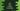# C# program to find a value in an array## C# array methods to find a value in an array:

In C#, we have a couple of methods defined in the Array class that can be used to find a value in an array. Following are these methods:

1. Array.Find()
2. Array.FindAll()
3. Array.FindLast()

In this post, we will learn how to use these methods with examples.

### Array.Find():

Array.Find is defined as below:

``public static T Find<T>(T[] arr, Predicate[T] p)``

It takes two parameters. The first one is the array where search is running. The second one is the Predicate, that is used to find an element in the array.

Let’s take a look at the below program:

``````using System;

public class Program
{
public static void Main()
{
string[] givenArray = {"one", "two", "three", "four", "five", "six"};

var found = Array.Find(givenArray,e => e == "six");

Console.WriteLine(found);
}
}``````

Here,

• We are using Array.Find to find a string in the array.
• It retuns the value if it is found in the array.
• The last line is printing the value.

If you run this program, it will print the below output:

``six``

### Array.FindAll():

Array.FindAll method returns all the strings that matches the specific condition. It is defined as below:

``public static T[] FindAll<T>(T[] arr, Predicate<T> p)``

Here,

• arr is a one-dimensional array to search.
• p is the predicate which can be a lambda expression. It returns all the elements that matches the predicate

For example, let’s take a look at the below program:

``````using System;

public class Program {

public static void Main(string[] args) {
string[] arr = {"one", "two", "three", "four", "five"};

string[] result = Array.FindAll(arr, e => e.StartsWith("t"));

Console.WriteLine(string.Join("\n", result));
}
}``````

Here,

• arr is the given array.
• result is the string array FindAll is returning. FindAll is finding all strings those are starting with t.
• The string array is holding all strings in the array those are starting with t. It will print the below output:
``````two
three``````

### Array.FindLast:

Array.FindLast method is used to find the last element that matches a given predicate. It is defined as below:

``public static T FindLast<T>(T[] arr, Predicate<T> p)``

Here,

• arr is the given array.
• p is the given predicate or a lambda function that is used to matches in the array and returns the last element.

Let’s take a look at the below example:

``````using System;

public class Program {

public static void Main(string[] args) {
string[] arr = {"one", "two", "three", "four", "five"};

string result = Array.FindLast(arr, e => e.StartsWith("t"));

Console.WriteLine(result);
}
}``````

Here,

• arr is the given array
• Using FindLast, we are finding the last element in the array. It is searching for the last element that starts with t. The last line is printing the result.

It will print:

``three``